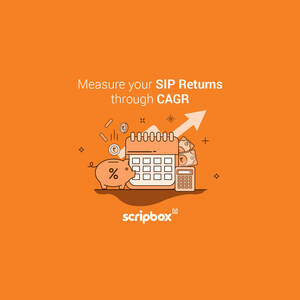# How should you really measure the rate of return on your SIP?

How you measure your rate of return can make a significant difference in terms of how you assess an investment.How you measure your rate of return can make a significant difference in terms of how you assess an investment. For mutual fund investors investing towards a goal, this is even more important. The rate of return that a goal demands, can decide whether you need to invest more or less to reach that goal.

What do you look for after you have figured out the financial goal and the asset class it will need (We hope you do that first!)?

The most likely answer is rate of return over multiple periods, and especially over extended periods.

However, many investors don’t see the same rate of return as the ones found on even the websites of mutual fund AMCs, much less in star ratings rankings that seem to change every year.

Investors are often puzzled by this. It’s much beyond “Past performance is not an indicator of future performance”. It’s more about the how and what.

The CAGR perspective

CAGR is one of the most popular ways in which returns from various asset classes is measured. Let’s consider the formula:

(Value of an investment at the end of the measurement period/ Value of an investment at the beginning of the measurement period)1/total period in years – 1

What this will tell you, based on the values you put in, is the returns from an investment from one period to another.

Let’s take the example of your investment in an FD. You invested Rs 1 Lakh on 3rd Jan 2015. On 3rd January 2019 you decided to close the FD as you needed the money for a short vacation. Let’s assume you got Rs 1.20 Lakh after tax.

On the surface it looks as if there was a 20% gain since the money grew by Rs 20,000. But we should not forget that this happened over a period of 4 years. The CAGR in this case using the formula shown, gives us 4.66%. So, your money grew by 4.66% each year.

This was calculated like this: (120,000/100000)^¼-1 = 4.66%

Similarly, when an equity mutual fund says it has delivered a CAGR of 16% since inception. This means that the fund grew by 16% annualised from its year of inception, say 1st January 2000 till 1st January 2019. For example, if you invested Rs 50,000 in 2000, it would have become Rs 8.4 Lakh by 2019.

But would your money have grown at the same pace had you invested in a SIP in the same mutual fund? Chances are not. Here’s why.

When you invest via SIP or even if you make periodic investments in a lump sum manner, each one of your investments grows at its own growth rate. Why? Simply because each investment has a different period that it has grown for.

Each SIP instalment is a separate investment

When you invest via SIP or even if you make periodic investments in a lump sum manner, each one of your investments grows at its own growth rate. Why? Simply because each investment has a different period that it has grown for.

Let’s consider a SIP of Rs 5000 started in January 2012. Till date (Jul 2019), you would have made a sum total of 91 SIP instalments. While the fund might have delivered 16% annualised between 2012 and 2019, you might have the same or a different rate of return.

The SIP instalment made in Jan 2012 would have grown the longest whereas the one made in July would have grown for the shortest period. Each SIP instalment would have a separate CAGR simply because the period for each would be different. This means that to arrive at a true picture of your growth you need to take into account the growth of each instalment.

Does this mean you’d have to do 91 CAGR calculations and aggregate them?

In a way yes, but there is a simpler way called XIRR. Using this approach, which is somewhat complex to do on paper, the modified CAGR is calculated for a set of investments across different periods. It is normally done using an application such as Microsoft Excel or Open Office Calc. XIRR in mathematical terms is nothing but the “weighted average” of multiple CAGRs. The approach also involves “guessing” the return for each investment to arrive at the actual rate of return for the whole investment.

Because of this you would arrive at a final return number that could be different than what the mutual fund shows. It could be higher or lower depending on the NAV value of the units you bought at the point. From the fund’s perspective, only the beginning value of the fund and the current value of the fund matters. But from your perspective, each of your instalment’s growth matters.

However, a consistent investment pattern over a long enough duration normally gives you a value which is more or less the same as the CAGR shown by the fund. This will rarely mean that you earn exactly the CAGR return mentioned by the fund. Therefore, take that rate of return only as indicative rather than an absolute certainty.

In the end, the only thing that matters is if you are on track towards your goal and is the rate of return overall enough to achieve this.